# Assignments

Differentiate the following functions.

1.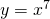Solution

2.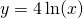Solution

3.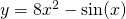Solution

4.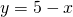Solution

5.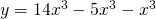Solution

6.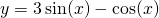Solution

7.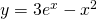Solution

8.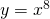Solution

9.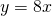Solution

10.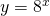Solution

11.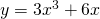Solution

12.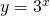Solution

13.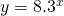Solution

14.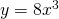Solution

15.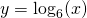Solution

16.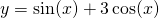Solution

17.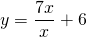Solution

18.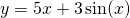Solution

19.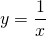Solution

20.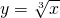Solution

21.Solution

22.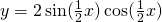Solution

23.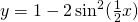Solution

24.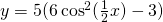Solution

25.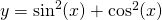Solution

26.Solution

27.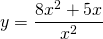Solution

28.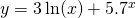Solution

29.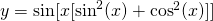Solution

30.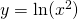Solution

0